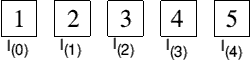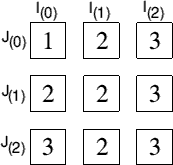C from the top

Part 6 - Arrays and more printing

The answers from last times puzzles are again too long to list here, so they are both on the cover disc again this month. As before though, a SAE with a floppy will get you them from myself or Archive.

More on printf

You will have already seen that by using the % sign preceded by a letter or number, that you can effect how items are displayed on the screen (a list was given in the second of these articles), however, you will have only seen following the \character one of two letters, either n or t.

You will have guessed that n is for a new line to be printed and t tabs along the text. There are still more of these, and are listed below

 Letter Meaning `\\` backslash `\'` single quote `\"` double quote `\?` question mark `\a` bell `\b` backspace `\f` form feed `\n` newline `\0` NULL `\r` carriage return `\t` tab `\v` vertical tab `\xhhh` insert ASCII code hhh

One addition to the % list given in the second article is %0. This causes all the leading zeros to be printed out.

That's all I need to add on the printf command, so on with arrays.

Arrays

An array can be thought of a set of boxes in memory, into which data can be stored. As with BASIC, there is no physical limit to the number of dimensions an array has, other than your machines memory.There is no DIM statement in C, arrays are defined by using the variable type, variable name and the number of component held within square brackets [].

```char string;
int fred;
double big;```

these define a character array of 30 elements, an integer of 35 and a double of 10.

An important fact to remember about arrays is that they go from 0 to n-1 (that is, if the array is , the array goes from 0 to 9).

If in BASIC, you wanted to define a DIM statement to contain some fixed data or other (say, birthdates), you would use something like this

```DIM birthdays(8)
FOR a%=1 TO 8
NEXT
DATA ........```

In C, this is done away with. To define a set of data elements for an array, you simply encase the data within a set of curly brackets.

`int birthdays  = { date1, date2, date3 .... };`

A two dimensional array works slightly differently as it has to be thought of as a block of x rowsThe above diagram should make it clearer. A 3 x 3 array contains 9 elements all told. The addressing of this array is as follows.

Say you want the 2nd element of the third row assigned to variable x, you would use

`x = array  ;`

remember, arrays go from 0, not from 1.

Defining a 2D array is the same as a 1D array, except the second element needs to be in held in another set of brackets

`int two_d  ;`

defines the int array two_d 10 elements by 10 elements (giving a total of 100 elements).

As before, the contents can be initialised by the use of curly brackets.

The 3D array is the same as a 2D, but with another set of numbers in square brackets.

`int three_d   ;`

This would give 5 down, by 10 across by 4 "diagonally". This gives a total of 5 x 10 x 4 = 200 elements.

Accessing a 3D array into a different variable (say you want point 3 - 6 - 2 - remembering that the array goes from 0 first though!)

`var = array   ;`

There are a couple of nice little tricks when dealing with arrays under C.

The first is when defining a 1D array and using a set of curly braces, you don't need to define the number of elements within the square braces - the compiler does this for you. This is known as an unsized array and is useful as you can always resize it should you need to. This can also be applied to a multi-dimension array. You don't need to define the first element, but do need to define the rest.

Strings need to be handled slightly differently.

`char names   ;`

this would mean we have 3 lists of strings (say names, birthdays and religion) of 6 element of strings, each of a maximum of 80 characters.

Accessing something from this array would be done by saying which list and which element

`printf("%s",names );`

would display the contents of the third persons religion.

Data can be entered into the array using the standard gets() function

`gets (name [list][element]);`

As you can by now appreciate, arrays can be very large (I have one program that creates an array of 1000 x 1000 x 1000, a total of 97.6Mb) and passing an array of this size across a number of functions really does slow even my RPC down (and worries the boots off the P3 it also runs on!).

A far simpler method (and much faster) of passing an array across multiple functions is to use a pointer.

This is defined in the same way as you would any other pointer

```int *array [x][y][z]; or

int array [x][y][z];

int *pointer;

pointer=array;```

this would define a pointer array of int values or a normal array, a pointer and then makes the pointer equal to the base of the array in memory.

Now, instead of passing over a huge array (or possibly a huge array!), you're now simply passing over a pointer containing the base of the array in memory. Far quicker!

There are a couple of drawbacks in using pointers with arrays. Firstly, look at the following snippet

```int x  = { 10 values };
int *pointer;
pointer=x;
printf("%d %d %d\n",*pointer,*(pointer+1),*(pointer+2));
printf("%d %d %d\n",x,x,x);```

You'll see at once that the pointer+1 is held within brackets. This is due to the pointer being a higher priority than the +. The brackets are a higher priority than the pointer, so this is processed first. Other than that, the two printf lines do exactly the same.

The second drawback is when accessing a multi-dimensioned array.

Say we've defined

`float numbers ;`

normally, we would access this by saying

`x = numbers ;`

however, we're using a pointer. The fragment would look like this

```float *pointer;
pointer = (float *) numbers;
printf("value at x = %d\n",*(pointer + (3 * 6) + 4);```

in other words, to access point , you first have to multiply the number of rows by the number of elements in that row (in this case, row 3 x 6 elements) then add the actual element you're after.

It is a lot of messing around and unless you're going to be passing this across a function, you're going to be better off the normal x = array ; command.

The (float*) is known as a cast and was needed in this example as the array had to be indexed manually so the pointer arithmetic had to be kept relative to a float pointer.

Character and numeric arrays can be also treated the same normal numbers and arrays within an if else or switch case condition.

That's enough for this time. Next time I'll be revisiting functions and looking at prototypes and recursion within a function. Until then, here's this times teasers....

1. Adjust the functions written in the simple maths program you should have done for this time so that the numbers can be floating point or integer and also that the sum and average function(s) use a dimension to store the numbers in (to a maximum of 10).

2. Write a phone directory program which stores the name, phone number (with the STD code separate), 4 lines for the address and 1 line for the postcode. Make sure this program has a lookup and display function so that you can find a name and number. The name and phone number arrays should be pointers. Make the program able to store 100 names.

This second program is far more important than the first and should be done as it will be built on later.